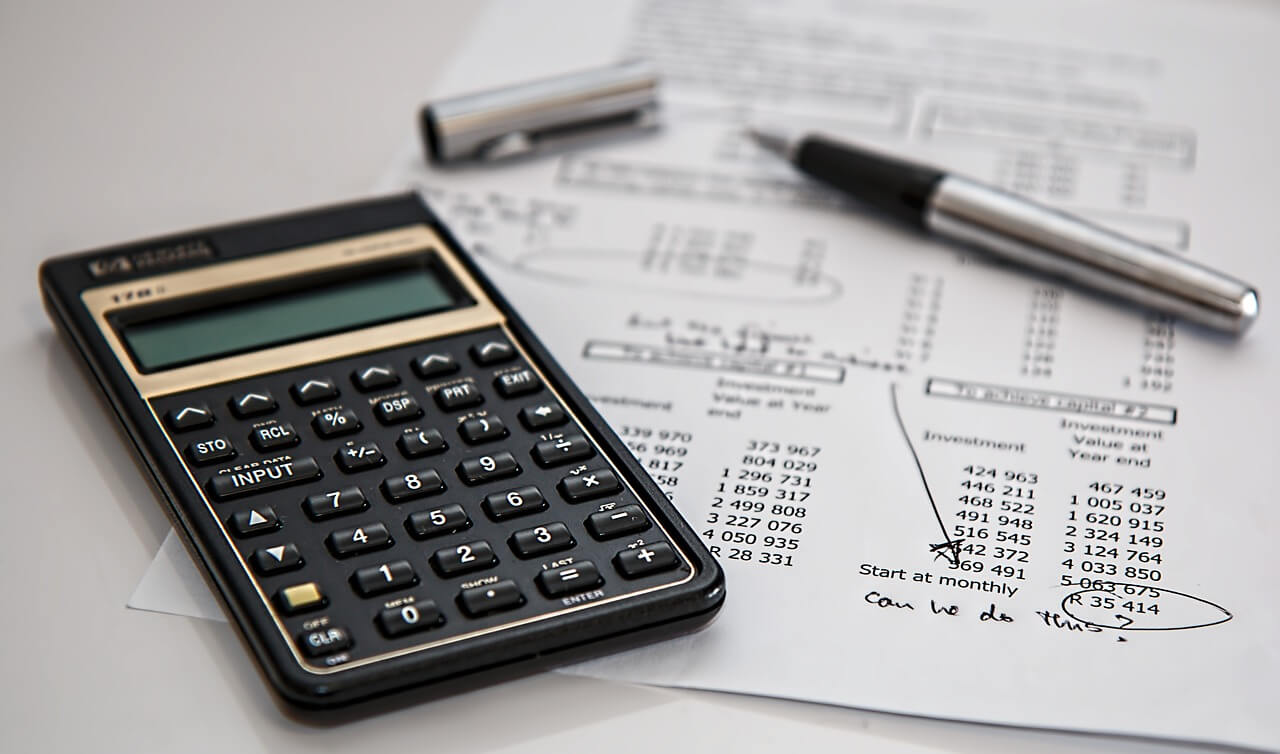# PERCENTAGE COUNTER PILKUNPIIPPITHE PERKIEST PERCENTAGE CALCULATOR ON THE INTERNET!

Pilkunpiippi brings colorful change to the old internet calculator offering and does not include any targeted advertising. With this percentage calculator, you can calculate the percentage of the difference between the figures, the percentage of increase and decrease, and VAT with ease.## Pilkunpiippi is much more than just a percentage calculator:

🔹 Subtract, add, and calculate the difference as a percentage

🔹 Change the color of the Pilkunpiippi to your liking

🔹 Hassle-free, worry-free and free!

🔹 More visually appealing than grey tables

🔹 This percentage calculator does not include targeted advertising

## CHECK OUT THE PERCENTAGE CALCULATOR PILKUNPIIPPI:Huomio! Koska prosenttilaskuri Pilkunpiippi on saatavilla pian myös ruotsin- ja englanninkielisenä, ei siinä ole asetettuna kiinteää verokantaa. Varmistathan siis kahdesti että prosenttiosuus on oikein kun lasket arvonlisäveroja. Suomessa alv-verokannat ovat 24%, 14% ja 10%. Useimpia tavaroita ja palveluita koskee niin sanottu yleinen verokanta, 24%.

Apuja arvonlisäveroasioihin löydät verottajan sivuilta.

••••••••••••## CALCULATION FORMULAS FOR THE PERCENTAGE CALCULATOR WITH EXAMPLE FIGURES:

When we want to know how much is 18% of 472:
The total number (in this case 472) is divided by 100 and then multiplied by a percentage.
(472 : 100) * 18 = 84,96.

To find out what 38% of the figure is 622:
622 is multiplied by 100 then divided by 38.
(622 * 100) : 38 = 1636,84.

When you want to know what percentage 63 is of 460:
63 is multiplied by 100 and the result is divided by the integer.
(63 * 100) : 460 = 13,7%

How much is the 20% discount on a product costing €490:
The price of the product is multiplied by the percentage of the discount, and the result is divided by 100.
The final result is deducted from the price of the product.
490 - ((490 * 20) : 100) = 392€.

How much will a service costing €325 cost when increased by 14%?:
Multiply the price of the product by 1.14. Of this figure, 1.0 is the starting price (100%) and 0.14 is the 14% mark-up.
325 * 1,14 = 370,5€.